# C语言博客作业04 数组

## 0.展示PTA总分（0----2)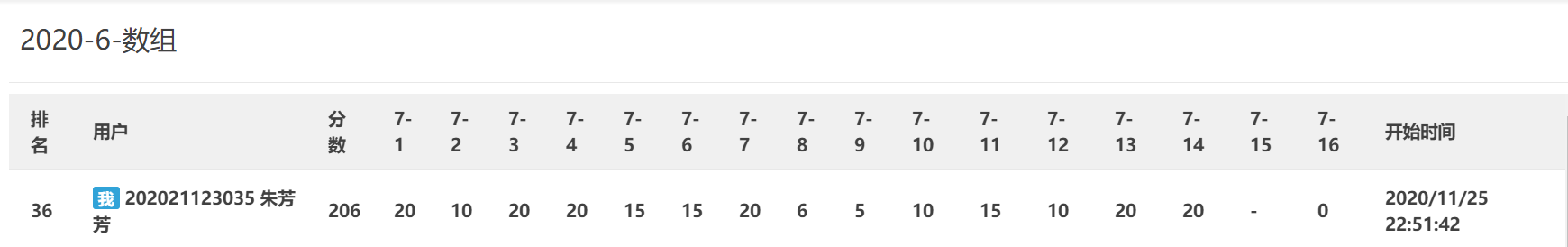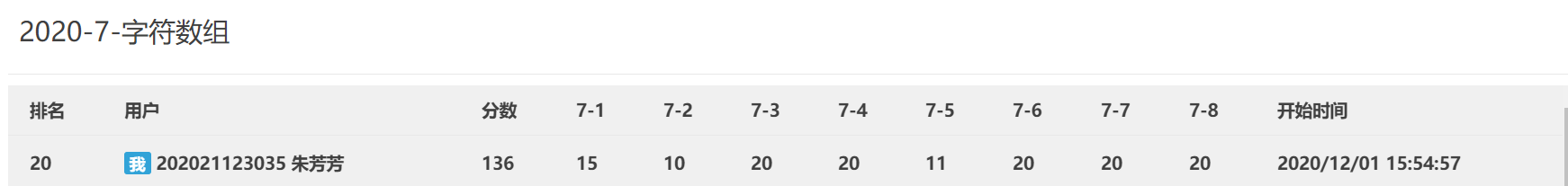## 1.本章学习总结（3分）

### 1.1.1知识点梳理

eg：
int score;
char score;

1、先声明，后赋值。

    类型 数组名[长度];
数组名[下标] = 值;


2、在声明的时候初始化。

    1)、类型 数组名[长度] = {数据1,数据2,数据3,数据4,.......};
eg：char  charTest = {'a','b','c'};
2)、类型 数组名[] = {数据1,数据2,数据3,数据4,.......};
eg：char  charTest[] = {'a','b','c'};
//第二种方式的长度，编译器会根据元素的个数来确定。
3)、类型 数组名 = {数据1,数据2};
//给的数据小于指定的长度
eg：char  charTest = {'a','b','c'};
4)、类型 数组名 = {[下标]=数据1,[下标]=数据2,};
//给的数据小于指定的长度
eg：char  charTest = { = 'a', = 'b', = 'c'};


   用循环依次访问数组中的每一个元素。


  定义：访问不属于数组本身空间的元素，运行时会报错，也可能是未知的异常。


  1 sizeof(数组名);：得到这个数组占用内存的总长度。
数组的长度 = sizeof(数组名) / sizeof(类型);
2引进库函数<string. h>,使用strlen();

1、数组和数组变量之间，不能赋值。
eg：
int nums1 = {1,2,3,4,5};
int nums2 = nums1;
2、数组名声明后也不能直接赋值。
eg：
int nums1 ;
nums1 = {1,2,3,4,5};
原因：数组名是一个地址常量。


**语法**：


void test1(int nums[]){
nums=11;
nums=22;
nums=33;
}
int main(int argc,const char * argv[])
{
int nums[] = {1,2,3};
test();
for(int i = 0;i<3;i++)
{
//注意：这里输出的不是1,2,3，而是11,22,33。
printf("%d \n",nums[i]);
}
return 0;
}

1、在函数中，不能直接计算形参(数组)的长度，永远等于2。解决办法就是在多传递一个参数，作为数组的长度。
2、函数的数组形参可以不写长度，但是区分类型。

### 1.1.2知识点技巧总结

• 顺序查找
• 二分查找
• 插值查找
• FIbonacci查找
• 分块查找
• 树表查找
• HashTable查找

eg: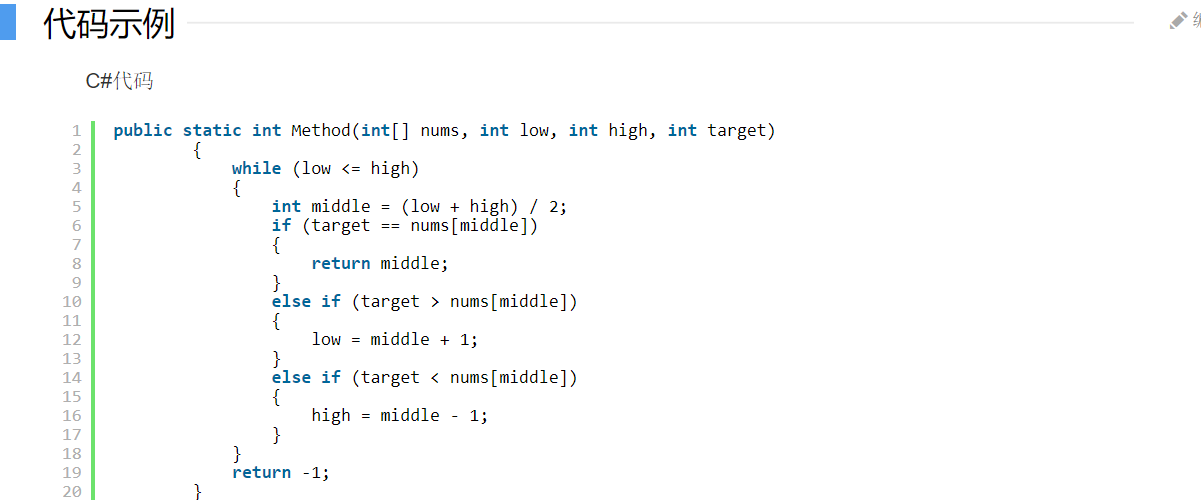while（移动次数大于0）
{
for()
}

#include<stdio.h>
#define N 10
int main ()
{
int a[N];
int i,j,t;
for(i<0;i<N;i++)
{
scanf("%d",&a[i]);
}
for(i=1;i<=N-1;i++)
{
for(j=0;j<N-i;j++)
{
if(a[j]>a[j+1])
{
t=a[j];a[j]=a[j+1];a[j+1]=t;
}
}
}
printf("The resortrd numbers is :\n");
for(i=0;i<N;i++)
{
printf("%-4d",a[i]);
}
}



//选择法：选择法的算法思想是先固定第一个值，将该固定的值依次与后面的值进行比较，如果大于/小于，则交换来达到将最大值或最小值放在最后。#include"stdio.h"
#include<stdio.h>
#define N 4
int main ()
{
int a[N];
int i,j,k,t;
for(i=0;i<N;i++)
{
scanf("%d",&a[i]);
}
printf("\n");
for(i=0;i<N;i++)
{
k=i;
for(j=i+1;j<N;j++)
{
if(a[k]>a[j])				//将两个数进行交换
{
k=j;
}
}
if(k!=i)
{
t=a[k];a[k]=a[i];a[i]=t;
}
}
for(i=0;i<N;i++)
{
printf("%d 	",a[i]);
}
}


#include <stdio.h>
#define N 5 //宏定义，并不是必须的
int main()
{
int powers[N] = {42332, 45771, 40907, 41234, 40767};
int count = 5;//元素个数
int deletePower;//用户要删除的元素
int deleteIndex = -1;//对应删除的元素下标,赋值一个不可能的值
int insertPower;
int i;

/*********数组的删除************/
printf("请输入要删除的元素：");
scanf("%d",&deletePower);
for (i = 0; i < count ;i++)
{
if(deletePower == powers[i])
{
deleteIndex = i;
break;
}
else
{
printf("您输入的值未在数组中，请重新输入！\n");
exit(0);
}

}
count--;
for(i = deleteIndex; i < count; i++)
{
powers[i] = powers[i+1];

}
printf("您删除后的数组为：");
for(i=0;i<count;i++)
{
printf("%d  ",powers[i]);
}
printf("\n");
/*********数组的插入************/
printf("请输入要插入的元素："); //默认插在数组最后
scanf("%d",&insertPower);
powers[count] = insertPower;
printf("您插入后的数组为：");
for(i=0;i<count+1;i++)
{
printf("%d  ",powers[i]);
}
}


1.构建一个新的数组存放结果

2.for循环中每次从原数组中取出一个元素，用这个元素循环与结果数组对比

3.若结果数组中没有该元素，则存到结果数组中

1.先将原数组进行排序

2.检查原数组中的第i个元素 与 结果数组中的最后一个元素是否相同，因为已经排序，所以重复元素会在相邻位置

3.如果不相同，则将该元素存入结果数组中

1.创建一个新的数组存放结果2.创建一个空对象3.for循环时，每次取出一个元素与对象进行对比，如果这个元素不重复，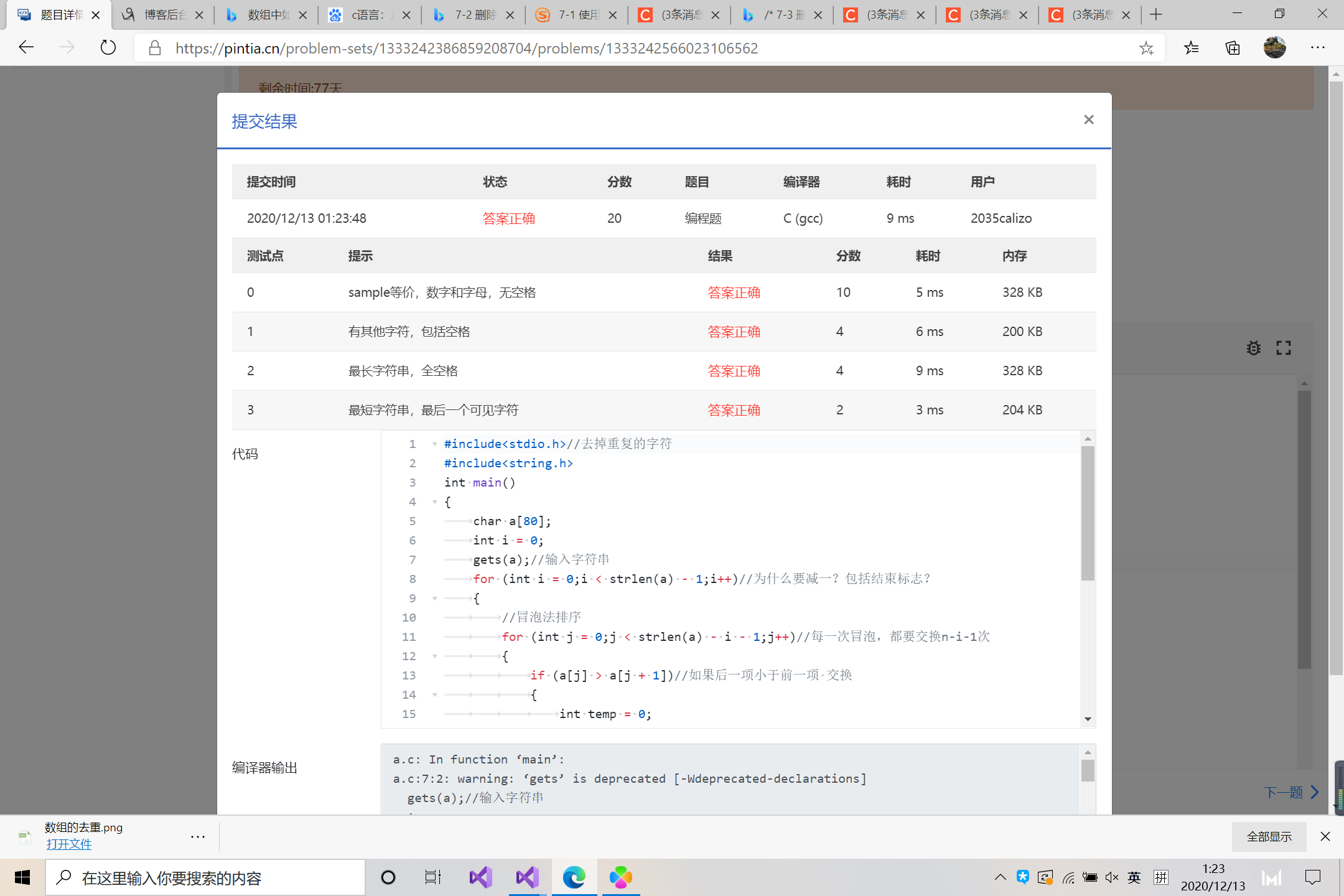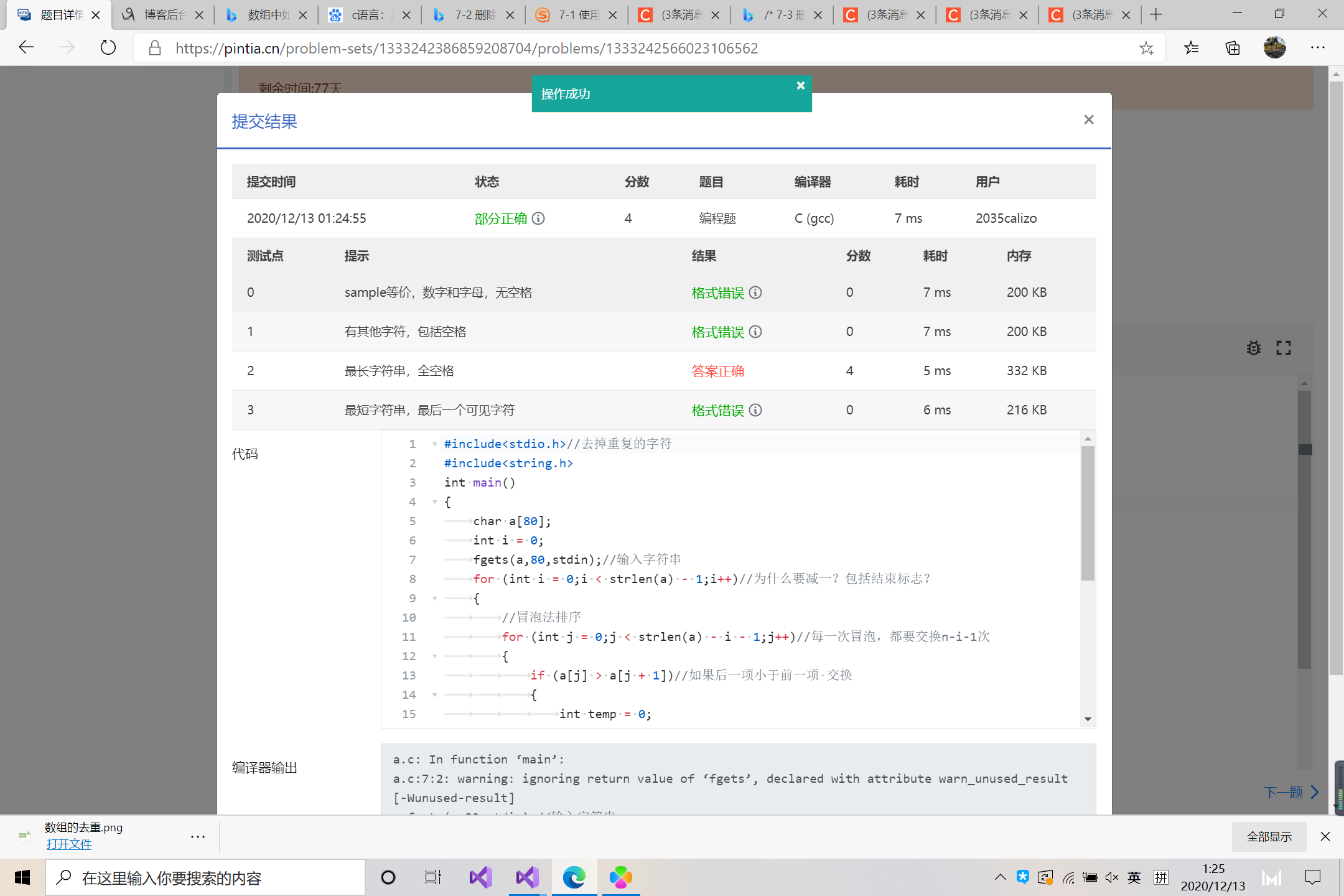## 2.PTA实验作业（7分）

### 2.1 冒泡法排序 （3分）

#### 2.1.1 伪代码

for(;i<k;)//外层循环，控制排序的次数k
{
for(；n-i-1；)//每一轮的排序，次数随着最大数放到最右边的个数而减少，与K没有直接联系
{

}//内层循环，控制每轮将最大的数放到最后
}
end for;
printf("%d ",a[i]);
for(int i=0;i<n-1;i++)//输出数组使用循环,控制格式对第一个单独处理
{
printf("%d",a[n-1]);
}
end for;

#### 2.1.2 代码截图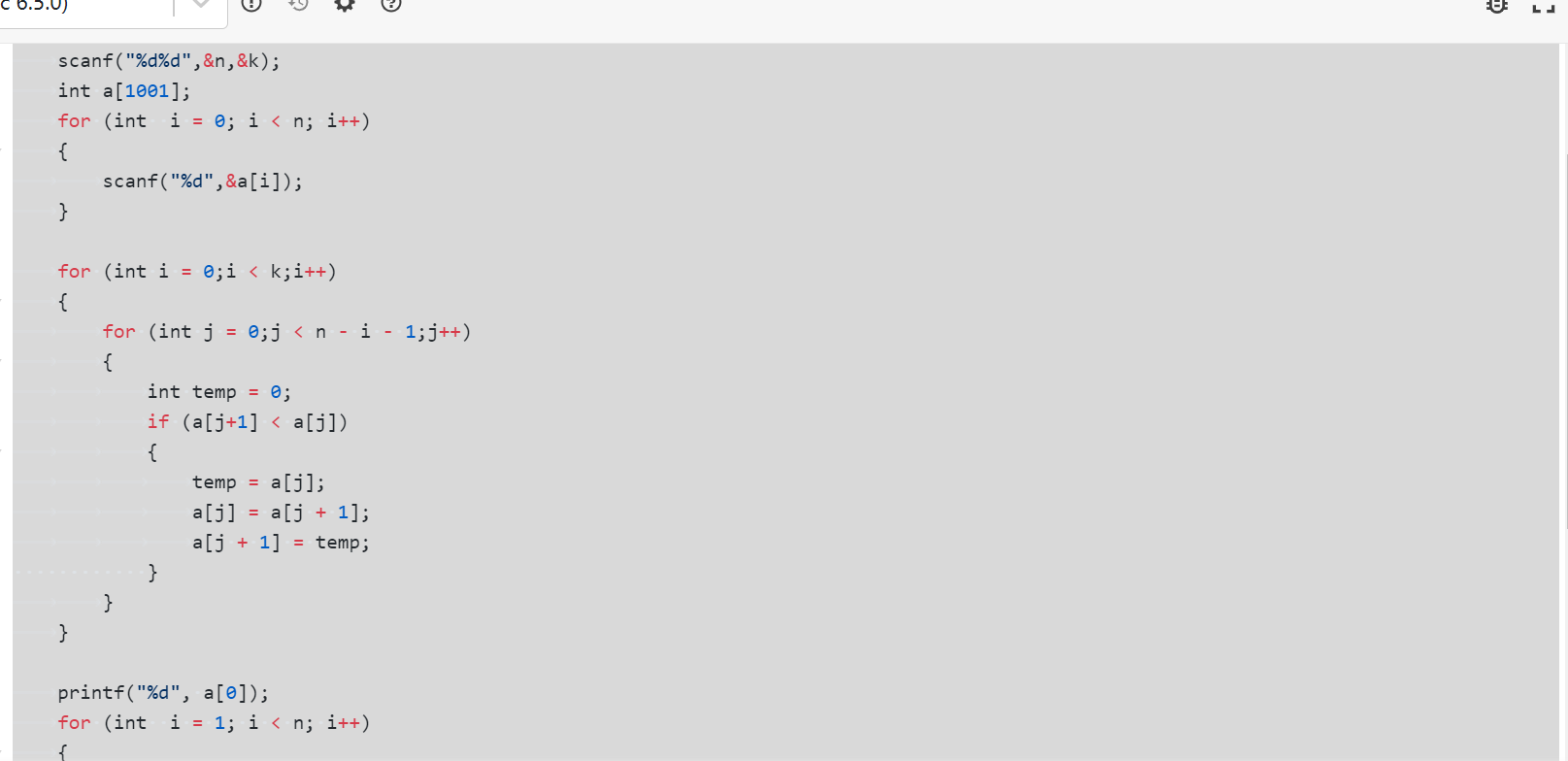### 2.2 找鞍点（2分）

for()
{

}

for()
{

}
for()
{

}

if（找到）
{

}
else
{

}

#### 2.2.2代码截图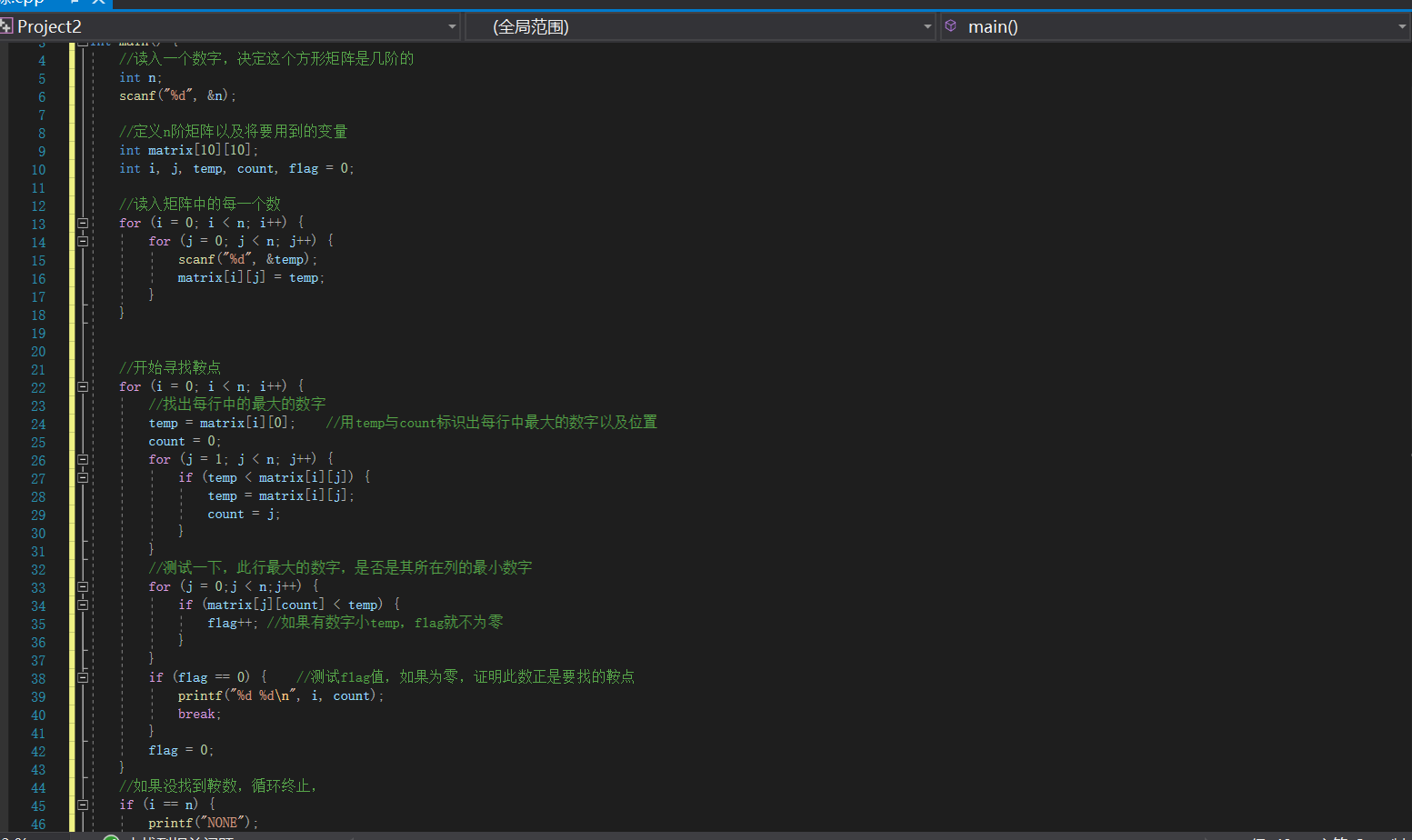### 2.3 切分表达式（2分）

while()//
{

}
end while;

#### 2.3.2 代码截图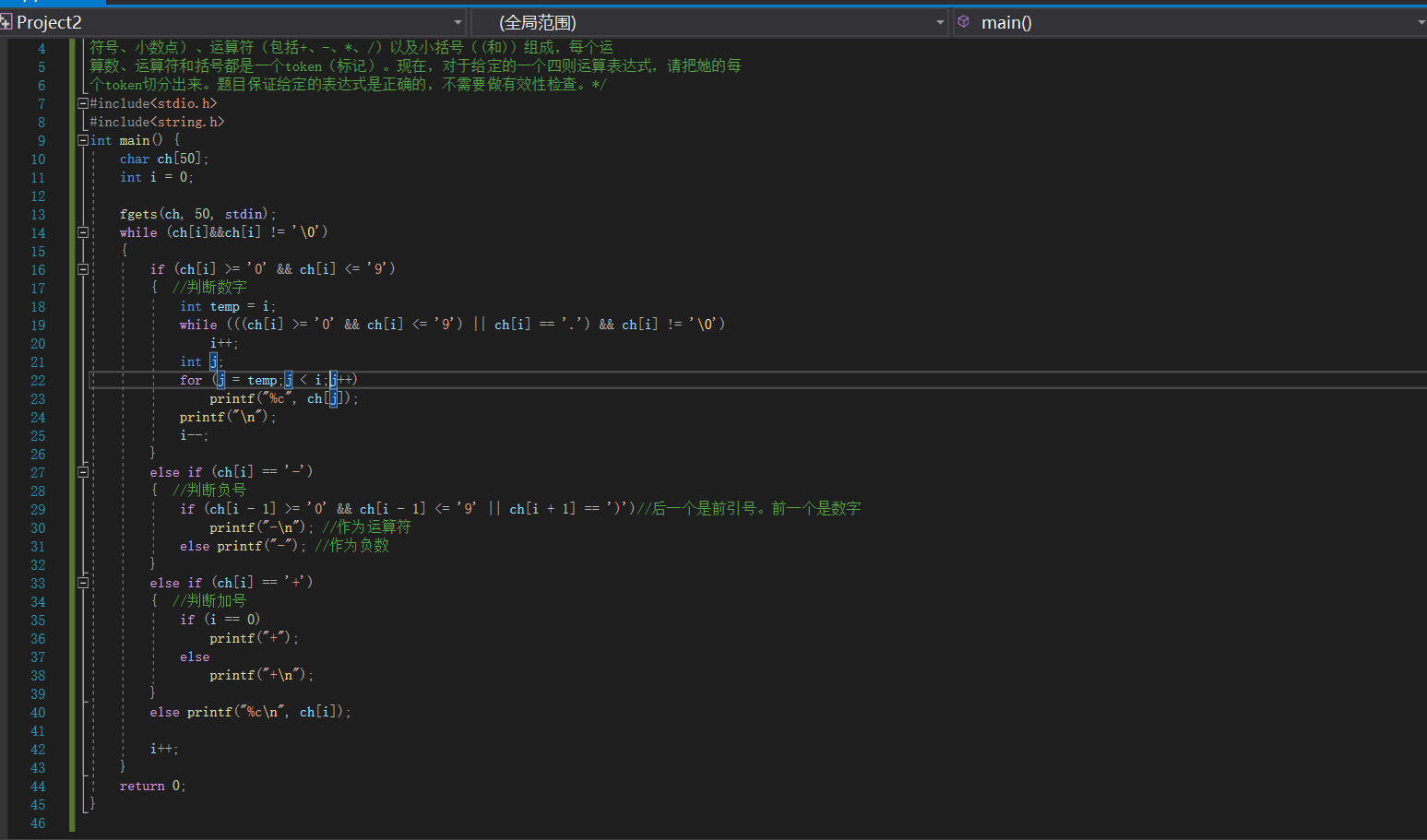#### 2.3.3

posted @ 2020-12-13 22:24  calizo  阅读(226)  评论(0编辑  收藏  举报